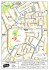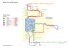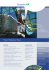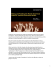# Vebra SOLEX

## Transcription

Vebra SOLEX
```89b Joel Street, Northwood HA6 1LU
Price Guide:
B E D R O O M 1
1 5 ' x 1 0 '4
4 .6 m x 3 .2 m
C U P B O A R D
guide price £149,950
R E C E P T IO N R O O M
1 5 ' x 1 0 '8
4 .6 m x 3 .2 m
C U P B O A R D
C U P B O A R D
B E D R O O M 2
1 2 '2 x 7 '
3 .7 m x 2 .1 m
E N T R A N C E
H A L L
T O T A L A P P R O X . F L O O R
W h ils t e v e r y a t te m p
o f d o o r s , w in d o w s ,
o m is s io n , o r m is - s
p r o s p e c t iv e p u r c h a s
K IT C H E N
1 3 '2 x 6 '1
4 .0 m x 1 .9 m
A R E A
5 9 9 S Q .F T . ( 5 5 .7 S Q .M .)
t h a s b e e n m a d e to e n s u r e t h e a c c u r a c y o f t h e flo o r p la n c o n t a in e
r o o m s a n d a n y o t h e r it e m s a r e a p p r o x im a t e a n d n o r e s p o n s ib il i t y
t a t e m e n t . T h is p la n is f o r illu s t r a t i v e p u r p o s e s o n ly a n d s h o u ld b e
e r . T h e s e r v ic e s , s y s t e m s a n d a p p lia n c e s s h o w n h a v e n o t b e e n t e
a s t o t h e ir o p e r a b il i t y o r e f f i c ie n c y c a n b e g iv e n
M a d e w ith M e tro p ix © 2 0 1 1
d h e re , m e a s u re m e n ts
is t a k e n f o r a n y e r r o r ,
u s e d a s s u c h b y a n y
s te d a n d n o g u a ra n te e
Lease approx 89 years remaining.
London Borough of Hillingdon
01923 833799
[email protected]
Viewing : Strictly through owners agents, Bennett Holmes (all sizes accurate
to plus or minus 5%).
A 2 bedroom top floor flat over shops in Northwood
Hills offered for sale in our opinion in good decorative
order. We are advised the lease is approximately 89
years remaining and has the benefit of NO UPPER
CHAIN. Ideally suited for investment buyers.
89b Joel Street
Northwood HA6 1LU
Price Guide:
guide price
£149,950
Inside
Accomodation comprises entrance hallway,
reception room, fitted kitchen, 2 bedrooms and
bathroom.
Outside
The property benefits from pavement access from
the shops.
.
.
```

### SH3 SH4 WW3 BT6 HA2 HA3 London Borough of Bromley Electoral### Buses from West Drayton